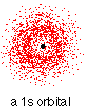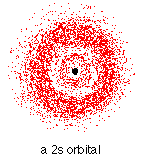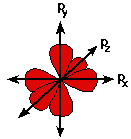# Orbitals

What is an Atomic Orbital?

Orbitals and Orbits

When the a planet moves around the sun, you can plot a definite path for it which is called an orbit. A simple view of the atom looks similar and you may have pictured the electrons as orbiting around the nucleus. The truth is different, and electrons in fact inhabit regions of space known as orbitals. If an electron is in a particular orbital it will have a particular definable energy.

Each orbital has a name.

The orbital occupied by the hydrogen electron is called a 1s orbital. The “1” represents the fact that the orbital is in the energy level closest to the nucleus. The “s” tells you about the shape of the orbital. s orbitals are spherically symmetric around the nucleus – in each case, like a hollow ball made of rather chunky material with the nucleus at its centre.

Hydrogen’s electron – the 1s orbitalThe orbital on the left is a 2s orbital. This is similar to a 1s orbital except that the region where there is the greatest chance of finding the electron is further from the nucleus – this is an orbital at the second energy level.

2s (and 3s, 4s, etc) electrons spend some of their time closer to the nucleus than you might expect. The effect of this is to slightly reduce the energy of electrons in s orbitals. The nearer the nucleus the electrons get, the lower their energy.

3s, 4s (etc) orbitals get progressively further from the nucleus.

p orbitalsNot all electrons inhabit s orbitals (in fact, very few electrons live in s orbitals). At the first energy level, the only orbital available to electrons is the 1s orbital, but at the second level, as well as a 2s orbital, there are also orbitals called 2p orbitals.

A p orbital is rather like 2 identical balloons tied together at the nucleus. The diagram on the right is a cross-section through that 3-dimensional region of space. Once again, the orbital shows where there is a 95% chance of finding a particular electron.

Unlike an s orbital, a p orbital points in a particular direction – the one drawn points up and down the page. At any one energy level it is possible to have three absolutely equivalent p orbitals pointing mutually at right angles to each other. These are arbitrarily given the symbols px, py and pz. The p orbitals at the second energy level are called 2px, 2py and 2pz. All levels except for the first level have p orbitals.d and f orbitals

In addition to s and p orbitals, there are two other sets of orbitals which become available for electrons to inhabit at higher energy levels. At the third level, there is a set of five d orbitals (with complicated shapes and names) as well as the 3s and 3p orbitals (3px, 3py, 3pz). At the third level there are a total of nine orbitals altogether.

Fitting electrons into orbitals

You can think of an atom as a very bizarre house (like an inverted pyramid!) – with the nucleus living on the ground floor, and then various rooms (orbitals) on the higher floors occupied by the electrons. On the first floor there is only 1 room (the 1s orbital); on the second floor there are 4 rooms (the 2s, 2px, 2py and 2pz orbitals); on the third floor there are 9 rooms (one 3s orbital, three 3p orbitals and five 3d orbitals); and so on. But the rooms aren’t very big . . . Each orbital can only hold 2 electrons.

A convenient way of showing the orbitals that the electrons live in is to draw “electrons-in-boxes”.

“Electrons-in-boxes”

Orbitals can be represented as boxes with the electrons in them shown as arrows. Often an up-arrow and a down-arrow are used to show that the electrons are in some way different. The diagram (not to scale) summarizes the energies of the orbitals up to the 4p level. Notice that the s orbital always has a slightly lower energy than the p orbitals at the same energy level, so the s orbital always fills with electrons before the corresponding p orbitals.The order of filling orbitals

Electrons fill low energy orbitals (closer to the nucleus) before they fill higher energy ones. Where there is a choice between orbitals of equal energy, they fill the orbitals singly as far as possible.